# Knowledge Base

## Solution 13062: Solving a System of Equations on the TI-89 Family, TI-92 Plus, and Voyage™ 200 Using the Gauss-Jordan method.

### How can I solve a system of equations on the TI-89 Family, TI-92 Plus, and Voyage 200 using the Gauss-Jordan method?

The TI-89 family, TI-92 Plus, and Voyage 200 graphing calculators do not have a one-key Gauss-Jordan method function. However, if you know what transformations you want to accomplish on a matrix, there are several commands available that allow the user to add or swap rows as it is done when using the Gauss-Jordan method.

Example: Solve the following system of equations:

3X + 5Y = 10
-2X + 4Y = 5

Solution:

The matrix for this example (consisting of the coefficients of the terms in the equations) is given below:

bb = [ [3 5 10] [-2 4 5 ]]

1. Enter the matrix bb into the Data/Matrix Editor Please note that on the TI-92 Plus and Voyage 200 please disregard pressing [ALPHA]:

• If the Apps Desktop is turned off press [APPS]  . If turned on press [APPS] to display the Apps Desktop, then select Data/Matrix Editor App and press [ENTER] .
• Press [►]  [▼] [▼].
• Press [B] [B] [▼].
• Press [ALPHA]  [▼].
• Press .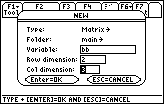• Press [ENTER] [ENTER].
• Press  [ENTER]  [ENTER]   [ENTER].
• Press [-]  [ENTER]  [ENTER]  [ENTER].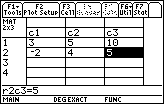2. Perform the row operations to put the matrix in reduced row echelon form:

• Press [HOME].
• Press [2ND] [MATH]  [ALPHA] [J] .
* Note: You can quickly access the command by pressing [CATALOG] and scrolling down to find it.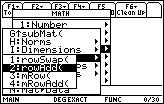• Press [ALPHA] [B] [ALPHA] [B] [,]  [,]  [)].
• Press [STO►] [ALPHA] [B] [ALPHA] [B].
• Press [ENTER].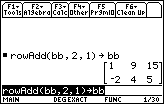• Press [2ND] [MATH]  [ALPHA] [J] .
• Press  [,] [ALPHA] [B] [ALPHA] [B] [,]  [,]  [)].
• Press [STO►] [ALPHA] [B] [B].
• Press [ENTER].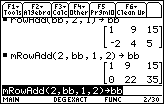• Press [2ND] [MATH]  [ALPHA] [J] .
• Press  [/]   [,] [ALPHA] [B] [ALPHA] [B] [,]  [)].
• Press [STO►] [ALPHA] [B] [ALPHA] [B].
• Press [ENTER].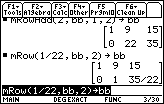• Press [2ND] [MATH]  [ALPHA] [J] .
• Press [-]  [,] [ALPHA] [B] [ALPHA] [B] [,]  [,]  [)].
• Press [STO►] [ALPHA] [B] [ALPHA] [B].
• Press [ENTER].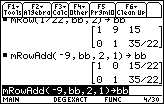The matrix is now in reduced row echelon form and the last column is the solution. The solutions are 15/22 for X and 35/22 for Y.

For additional information on using matrix operations and other functions on the TI-89 family, TI-92 Plus, and Voyage 200 refer to the online guidebooks.